# ISNA function in Excel with formula examples

This tutorial dives into various ways of using the ISNA function in Excel to handle #N/A errors.

When Excel cannot find what it is asked for, a #N/A error appears in a cell. To intercept and handle such errors, you can use the ISNA function. What's the practical use of that? Essentially, it helps to make your formulas more user-friendly and your worksheets better-looking.

## ISNA function in Excel

The Excel ISNA function is used to check cells or formulas for #N/A errors. The result is a logical value: TRUE if a #N/A error is detected, FALSE otherwise.

The function is available in all versions of Excel 2000 through 2021 and Excel 365.

The syntax of the ISNA function is as simple as it could possibly be:

ISNA(value)

Where value is the cell value or formula you want to check for #N/A errors.

To create an ISNA formula in its basic form, supply a cell reference as its only argument:

`=ISNA(A2)`

In case the referenced cell contains a #N/A error, you'll get TRUE. In case of any other error, value or a blank cell, you'll get FALSE: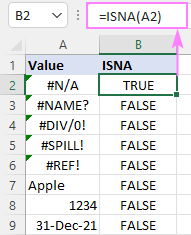## How to use ISNA in Excel

Using the ISNA function in its pure form has little practical sense. More often, it is used together with other functions to evaluate the result of a certain formula. For this, just put that other formula in the value argument of ISNA:

ISNA(your_formula())

In the below dataset, suppose you want to compare two lists (columns A and D) and identify the names that are present in both lists and those that appear only in list 1.

To compare the name in A3 against each name in column D, the formula is:

`=MATCH(A3, \$D\$2:\$D\$9, 0)`

If a lookup value is found, the MATCH function returns its relative position in the lookup array, otherwise a #N/A error occurs. To test the result of MATCH, we nest it in ISNA:

`=ISNA(MATCH(A3, \$D\$2:\$D\$9, 0))`

This formula goes to B3, and then is copied through B14.

Now, you can clearly see which students have passed all the tests (a name is not available in column D > MATCH returns #N/A > ISNA returns TRUE) and which have at least one failed test (a name appears in column D > no error > ISNA returns FALSE).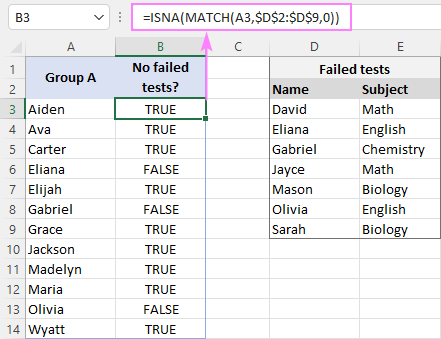Tip. In Excel 365 and Excel 2021, you can use a more modern XMATCH function. instead of MATCH.

## IF ISNA formula in Excel

By design, the ISNA function can only return two Boolean values. To display your custom messages, use it in combination with the IF function:

IF(ISNA(…), "text_if_error", "text_if_no_error")

Refining our example a little further, let's find out which students from group A did not fail any test and return "No failed tests" for them. For the remaining students, we'll return "Failed". To do this, embed the ISNA MATCH formula in the logical test of IF, so that IF becomes the outermost function:

`=IF(ISNA(MATCH(A3,\$D\$2:\$D\$9,0)), "No failed tests", "Failed")`

The results look much better and more intuitive now, agree?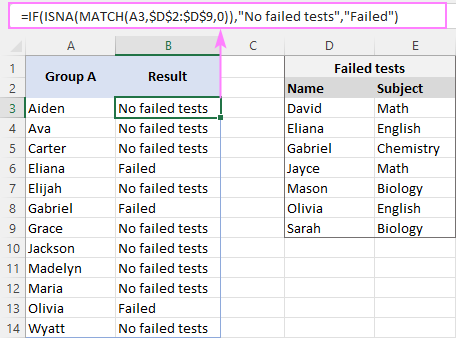## How to use ISNA in Excel with VLOOKUP

The IF ISNA combination is a universal solution that can be used with any function that searches for something in a set of data and returns a #N/A error when a lookup value is not found.

The syntax of the ISNA function with VLOOKUP is as follows:

IF(ISNA(VLOOKUP(…), "custom_text", VLOOKUP(…))

Translated into a human language, it says: if VLOOKUP results in a #N/A error, return custom text, otherwise return VLOOKUP's result.

In our sample table, assume you wish to return the subjects in which students failed tests. For those who have passed all the tests successfully, "No failed tests" is going to be displayed.

To look up the subjects, we construct this classic VLOOKUP formula:

`=VLOOKUP(A3, \$D\$3:\$E\$9, 2, FALSE)`

And then nest it in the generic IF ISNA formula discussed above:

`=IF(ISNA(VLOOKUP(A3, \$D\$3:\$E\$9, 2, FALSE)), "No failed tests", VLOOKUP(A3, \$D\$3:\$E\$9, 2, FALSE))`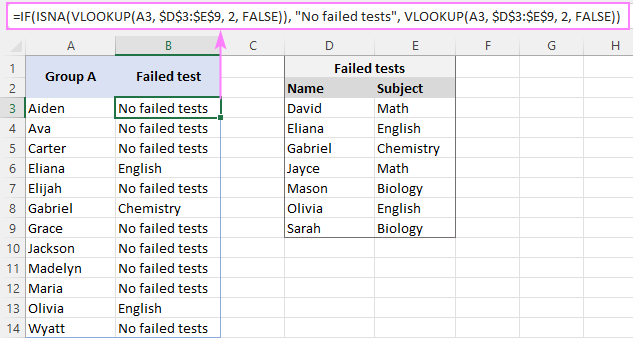In Excel 2013 and later version, you can utilize the IFNA function to catch and handle #N/A errors. This makes your formula shorter and easier to read.

As an example, we replace #N/A errors with dashes ("-") and get this elegant solution:

`=IFNA(VLOOKUP(A3, \$D\$3:\$E\$9, 2, FALSE), "-")`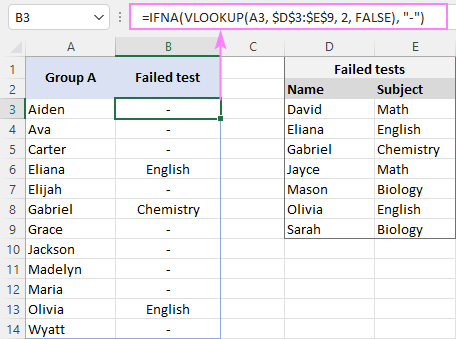The users of Excel 365 and 2021 don't need any wrapper function at all as the modern successor of VLOOKUP, the XLOOKUP function, can handle #N/A errors natively:

`=XLOOKUP(A3, \$D\$3:\$D\$9, \$E\$3:\$E\$9, "-")`

The result will be exactly the same as shown in the screenshot above.

## SUMPRODUCT ISNA formula to count #N/A errors

To count #N/A errors in a certain range, use the ISNA function together with SUMPRODUCT in this way:

SUMPRODUCT(--ISNA(range))

Here, ISNA returns an array of TRUE and FALSE values, the double negation (--) coerces the logical values into 1's and 0's, and SUMPRODUCT adds up the result.

For instance, to find out how many students succeeded in all tests, modify the MATCH formula for a range of lookup values (A3:A14) and nest it in ISNA:

`=SUMPRODUCT(--ISNA(MATCH(A3:A14, D2:D9, 0)))`

The formula determines that 9 students have no failed tests, i.e. the MATCH function returns 9 #N/A errors: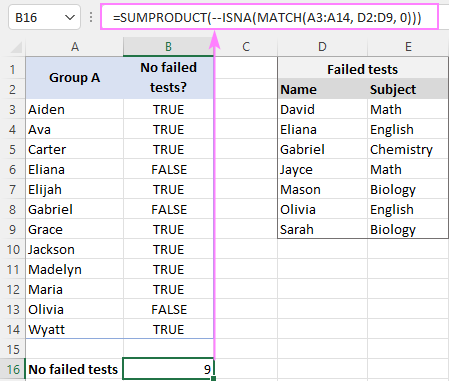That's how to create and use ISNA formulas in Excel. I thank you for reading and look forward to seeing you on our blog next week!

ISNA formula examples (.xlsx file)